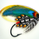‎1317‎ مشاهدة
1317
Daily and weekly fibo and traditional pivots in one scriptI've managed to add those lines and also deleted other pivots than weekly fibo ones...I miss two more features:
1 - be able to set how many periods back want to see (or at least have just current week)
2 - plot labels on each pivot line

Thanks fr you answer on private messages Segko!!!

//Created by Sergko13 18/02/2017 with Special Thanks to ChrisMoody
study(title="Daily and Weekly Pivots", shorttitle="DWP", overlay=true)

// Pivot
pivot = (high + low + close ) / 3.0

// Fibonacci Pivots
fr1 = pivot + (high - low) * 0.382
fs1 = pivot - (high - low) * 0.382
fr2 = pivot + (high - low) * 0.618
fs2 = pivot - (high - low) * 0.618
fr3 = pivot + (high - low)
fs3 = pivot - (high - low)
fra = pivot + (high - low) * 0.786
fsa = pivot - (high-low) * 0.786
frb = pivot + (high - low) * 1.382
fsb = pivot - (high-low) * 1.382
frc = pivot + (high - low) * 1.618
fsc = pivot - (high-low) * 1.618
frd = pivot + (high - low) * 2.00
fsd = pivot - (high-low) * 2.00

//Weekly Pivots
wtime_pivot = security(tickerid, 'W', pivot)
wtime_FR1 = security(tickerid, 'W', fr1)
wtime_FS1 = security(tickerid, 'W', fs1)
wtime_FR2 = security(tickerid, 'W', fr2)
wtime_FS2 = security(tickerid, 'W', fs2)
wtime_FR3 = security(tickerid, 'W', fr3)
wtime_FS3 = security(tickerid, 'W', fs3)
wtime_FRA = security(tickerid, 'W', fra)
wtime_FSA = security(tickerid, 'W', fsa)
wtime_FRB = security(tickerid, 'W', fra)
wtime_FSB = security(tickerid, 'W', fsa)
wtime_FRC = security(tickerid, 'W', fra)
wtime_FSC = security(tickerid, 'W', fsa)
wtime_FRD = security(tickerid, 'W', fra)
wtime_FSD = security(tickerid, 'W', fsa)

plot(wtime_pivot, title="Weekly Pivot", style=line, color=black,linewidth=1)
plot(wtime_FR1, title="Weekly Fibo R38",style=line, color=black,linewidth=1)
plot(wtime_FS1, title="Weekly Fibo S38",style=line, color=black,linewidth=1)
plot(wtime_FR2, title="Weekly Fibo R61",style=line, color=black,linewidth=1)
plot(wtime_FS2, title="Weekly Fibo S61",style=line, color=black,linewidth=1)
plot(wtime_FR3, title="Weekly Fibo R100",style=line, color=black,linewidth=1)
plot(wtime_FS3, title="Weekly Fibo S100",style=line, color=black,linewidth=1)
plot(wtime_FRA, title="Weekly Fibo R78",style=line, color=red,linewidth=1)
plot(wtime_FSA, title="Weekly Fibo S78",style=line, color=red,linewidth=1)
plot(wtime_FRB, title="Weekly Fibo R138",style=line, color=red,linewidth=1)
plot(wtime_FSB, title="Weekly Fibo S138",style=line, color=red,linewidth=1)
plot(wtime_FRC, title="Weekly Fibo R161",style=line, color=red,linewidth=1)
plot(wtime_FSC, title="Weekly Fibo S161",style=line, color=red,linewidth=1)
plot(wtime_FRD, title="Weekly Fibo R200",style=line, color=red,linewidth=1)
plot(wtime_FSD, title="Weekly Fibo S200",style=line, color=red,linewidth=1)
ردHi there,

Thanks for the script. I'm using the weakly fibo option and also wondering if you can add also R & S levels for 0.786, 1, 1.382, 1.618 and 2.00 fibo levels

Labels for each level line will also help.

Thanks!
رد